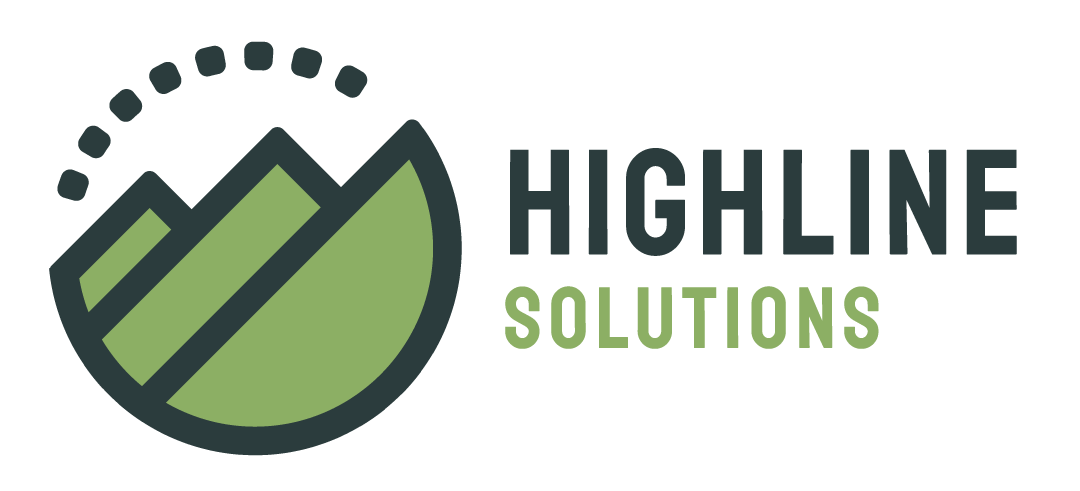# EPC Construction Rules

April 22nd 2011

Points
1. Let a be a point [distinct from . . . ].
2. Let a be a point on L [distinct from . . . ].
3. Let a be a point on L between b and c [distinct from . . . ].
4. Let a be a point on L extending the segment from b to c [with a distinct from. . . ].
5. Let a be a point on the same side of L as b [distinct from. . . ].
6. Let a be a point on the side of L opposite b [distinct from. . . ].
7. Let a be a point on a [distinct from . . . ].
8. Let a be a point inside a [distinct from . . . ].
9. Let a be a point outside a [distinct from . . . ].
Lines and Circles
1. Let L be the line through a and b [distinct from . . . ].
2. Let a be the circle with center a passing through b [distinct from . . . ].
Intersections
1. Let a be a point of intersection of L and M [distinct from . . . ].
2. Let a be a point of intersection of C and L [distinct from . . . ].
3. Let a and b be the two points of intersection of C and L [distinct from . . . ].
4. Let a be a point of intersection of L and C between b and c [distinct from . . . ].
5. Let a be a point of intersection of L and C extending the segment from c to b [distinct from . . . ].
6. Let a be a point of intersection of C and D [distinct from . . . ].
7. Let a and b be the two points of intersection of C and D [distinct from . . . ].
8. Let a be a point of intersection of C and D on the same side of L as b where L is the line through their centers c and d respectively [distinct from . . . ].
9. Let a be a point of intersection of C and D on the side of L opposite b where L is the line through their centers c and d respectively [distinct from . . . ].I'm an "old" programmer who has been blogging for almost 20 years now. In 2017, I started Highline Solutions, a consulting company that helps with software architecture and full-stack development. I have two degrees from Carnegie Mellon University, one practical (Information and Decision Systems) and one not so much (Philosophy - thesis here). Pittsburgh, PA is my home where I live with my wife and 3 energetic boys.We're hiring! Looking for a full-stack developer (React, NodeJS or Java, AWS) open to contract work. Fully remote. The model at Highline is a little different - we're more of a co-op than a traditional consultancy. Our goal is to reward the person doing the work, and keep everything else streamlined. If you're interested, hit me up at ben@highlinesolutions.io to find out more. Send along a resume, or better yet a TechRez!I recently released a web app called TechRez, a "better resume for tech". The idea is that instead of sending out the same-old static PDF resume that's jam packed with buzz words and spans multiple pages, you can create a TechRez, which is modern, visual, and interactive. Try it out for free!